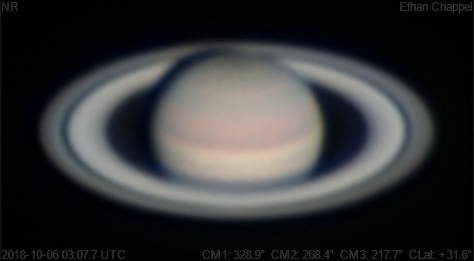# Saturn 2018-10-06 03:07 UTC

CM1: 328.90°

CM2: 268.40°

CM3: 217.70°

CLat: +31.60°

Description

Seeing was not as cooperative for the first time in a few months. I decided to overhaul my imaging train for the C8. The ASI224MC has been swapped out for my ASI174MM with Orion RGB filters. While it takes work to get reasonably accurate colors with these filters, I find satisfaction with the colors captured by OSC cameras to be rare. Since the ASI74MM has larger pixels, I've switched back to the Celestron X-Cel 3x barlow to compensate.

Equipment

ZWO ASI174MM

Celestron X-Cel 3x

Celestron EdgeHD 8

Logs
```FireCapture v2.6  Settings
------------------------------------
Observer=Ethan Chappel
Camera=ZWO ASI174MM
Filter=B
Profile=Saturn
Diameter=16.25"
Magnitude=0.55
CMI=331.3° CMIII=219.9°  (during mid of capture)
FocalLength=8500mm (F/23)
Resolution=0.14"
Filename=2018-10-06-0311_7-EC-B-Sat.ser
Date=2018_10_06
Start=03_10_30.869
Mid=03_11_45.874
End=03_13_00.879
Start(UT)=03_10_30.869
Mid(UT)=03_11_45.874
End(UT)=03_13_00.879
Duration=150.010s
Date_format=yyyy_MM_dd
Time_format=HH_mm_ss
LT=UT -6h
Frames captured=2142
File type=SER
Binning=no
Bit depth=8bit
Debayer=no
ROI=332x194
ROI(Offset)=0x0
FPS (avg.)=14
Shutter=70.00ms
Gain=400 (100%)
AutoHisto=75 (off)
FPS=100 (off)
AutoExposure=off
SoftwareGain=10 (off)
Brightness=1 (off)
Gamma=50 (off)
HighSpeed=off
AutoGain=off
USBTraffic=80
Histogramm(min)=0
Histogramm(max)=255
Histogramm=100%
Noise(avg.deviation)=2.67
AutoAlign=false
PreFilter=none
Limit=150 Seconds
Sensor temperature=39.2°C
FireCapture v2.6  Settings
------------------------------------
Observer=Ethan Chappel
Camera=ZWO ASI174MM
Filter=G
Profile=Saturn
Diameter=16.25"
Magnitude=0.55
CMI=328.9° CMIII=217.6°  (during mid of capture)
FocalLength=8500mm (F/23)
Resolution=0.14"
Filename=2018-10-06-0307_7-EC-G-Sat.ser
Date=2018_10_06
Start=03_06_29.117
Mid=03_07_45.666
End=03_09_02.215
Start(UT)=03_06_29.117
Mid(UT)=03_07_45.666
End(UT)=03_09_02.215
Duration=153.098s
Date_format=yyyy_MM_dd
Time_format=HH_mm_ss
LT=UT -6h
Frames captured=2550
File type=SER
Binning=no
Bit depth=8bit
Debayer=no
ROI=332x194
ROI(Offset)=0x0
FPS (avg.)=16
Shutter=60.00ms
Gain=400 (100%)
AutoHisto=75 (off)
FPS=100 (off)
AutoExposure=off
SoftwareGain=10 (off)
Brightness=1 (off)
Gamma=50 (off)
HighSpeed=off
AutoGain=off
USBTraffic=80
Histogramm(min)=0
Histogramm(max)=255
Histogramm=100%
Noise(avg.deviation)=2.63
AutoAlign=false
PreFilter=none
Limit=none
Sensor temperature=38.7°C
FireCapture v2.6  Settings
------------------------------------
Observer=Ethan Chappel
Camera=ZWO ASI174MM
Filter=R
Profile=Saturn
Diameter=16.25"
Magnitude=0.55
CMI=326.5° CMIII=215.4°  (during mid of capture)
FocalLength=8500mm (F/23)
Resolution=0.14"
Filename=2018-10-06-0303_7-EC-R-Sat.ser
Date=2018_10_06
Start=03_02_28.351
Mid=03_03_43.372
End=03_04_58.393
Start(UT)=03_02_28.351
Mid(UT)=03_03_43.372
End(UT)=03_04_58.393
Duration=150.042s
Date_format=yyyy_MM_dd
Time_format=HH_mm_ss
LT=UT -6h
Frames captured=2247
File type=SER
Binning=no
Bit depth=8bit
Debayer=no
ROI=332x194
ROI(Offset)=0x0
FPS (avg.)=14
Shutter=66.76ms
Gain=400 (100%)
AutoHisto=75 (off)
FPS=100 (off)
AutoExposure=off
SoftwareGain=10 (off)
Brightness=1 (off)
Gamma=50 (off)
HighSpeed=off
AutoGain=off
USBTraffic=80
Histogramm(min)=0
Histogramm(max)=255
Histogramm=100%
Noise(avg.deviation)=2.63
AutoAlign=false
PreFilter=none
Limit=150 Seconds
Sensor temperature=36.8°C
```